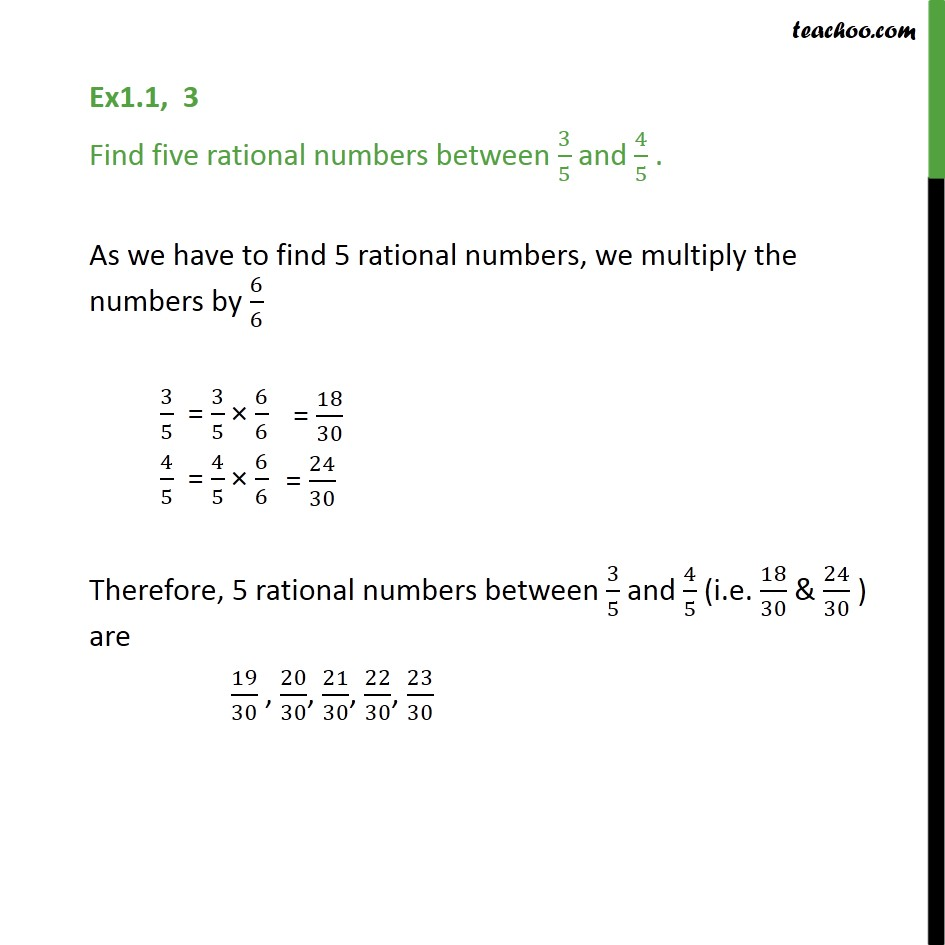1. Chapter 1 Class 9 Number Systems (Term 1)
2. Serial order wise
3. Ex 1.1

Transcript

Ex1.1, 3 Find five rational numbers between 3/5 and 4/5 . As we have to find 5 rational numbers, we multiply the numbers by 6/6 3/5 = 3/5 × 6/6 4/5 = 4/5 × 6/6 Therefore, 5 rational numbers between 3/5 and 4/5 (i.e. 18/30 & 24/30 ) are 19/30 , 20/30, 21/30, 22/30, 23/30